## Product Management / Product Ownership Training

Entdecke die Welt des effektiven Product Managements. Nächster Trainingstermin: 11.-14.12. (Remote) – jetzt buchen!
Zum TrainingData Science

# Dimensionality Reduction with Principal Component Analysis

Autor:in
Lesezeit
13 ​​min

This article takes a look at principal component analysis (PCA). In the course of the article, we will work our way through the most important theoretical knowledge and create an implementation of PCA in Python.

The content of this blog post was originally published as a Jupyter notebook here. You can run the code interactively in your browser using Binder.

## What is principal component analysis?

In simple terms, principal component analysis (PCA) is a technique to perform dimensionality reduction. It has been around for more than 100 years and is still heavily used. Although PCA is most often applied to find a lower-dimensional representation of data, it can also be used for other purposes, e.g., to detect simple patterns in data.

## Why do we need principal component analysis?

A lot of data is high-dimensional. Imagine patient data collected in a hospital. For each patient, we can have hundreds or even thousands of measurements (blood pressure, heart rate, respiratory rate, etc.). Working with such data is difficult – it is expensive to store, hard to analyze, and almost impossible to visualize.

Luckily, many dimensions in high-dimensional data are often redundant and can be expressed by combining some of the other dimensions. Also, the key part of the data is often contained in a more compact lower-dimensional structure. Consequently, we can simplify high-dimensional data points using dimensionality reduction techniques like principal component analysis (PCA).

## Key ideas of PCA

• PCA finds a lower-dimensional representation of data by constructing new features (called principal components) which are linear combinations of the original features
• The new features are selected carefully: PCA looks for features that can summarize the data best without losing too much information

## Two ways of deriving PCA

Let’s say we have an i.i.d dataset $$\boldsymbol{X}$$ with $$D$$ dimensions and a mean value of $$0$$:
$$\mathcal{\boldsymbol{X}} = \{\boldsymbol{x}_1, …, \boldsymbol{x}_N\} \boldsymbol{x}_n \in \mathbb{R}^D$$. The data covariance matrix (which will be needed later on) is computed as follows:

$$\boldsymbol{S} = \frac{1}{N} \sum_{n=1}^{N} \boldsymbol{x}_n \boldsymbol{x}_n^T$$

In PCA our goal is to find projections of the datapoints $$\boldsymbol{x}_n$$ that are as similar to the original data points as possible but have lower dimensionality. We can approach this goal from two perspectives:

1. Maximum variance perspective: We try to find a low-dimensional representation that maximizes the variance of the projected data.
2. Projection perspective: We try to find a low-dimensional representation that minimizes the average reconstruction error between the original data and the reconstructed data.

Both approaches reach the same result.

### Maximum variance perspective

In the maximum variance perspective, PCA is derived as an algorithm that tries to find a transformation matrix $$\boldsymbol{B}$$ which projects the original data $$\boldsymbol{X}$$ to a low-dimensional space, ideally without losing information. Let’s say that this low-dimensional space has dimension $$M$$.

How can we make sure that we find a matrix $$B$$ that retains as much information as possible? It turns out that this is achieved when $$\boldsymbol{B}$$ projects the data in a way that maximizes the variance of the data in the (new) low-dimensional space.

Let’s take a closer look at $$\boldsymbol{B}$$. We can define $$\boldsymbol{B}$$ as a collection of vectors $$\boldsymbol{b}_1, …, \boldsymbol{b}_M$$:

$$\boldsymbol{B} := [\boldsymbol{b}_1, …, \boldsymbol{b}_M] \in \mathbb{R}^{D x M}$$

The vectors $$\boldsymbol{b}_m$$ are called basis vectors and form the axes of the new $$M$$-dimensional space we project our data to.

When deriving which vectors $$\boldsymbol{b}_m$$ we should select to maximize the variance, we will find that maximizing the variance is equivalent to selecting those vectors that belong to the largest eigenvalues of the data covariance matrix $$\boldsymbol{S}$$. That means that we can construct our matrix $$\boldsymbol{B}$$ by first computing the eigenvalues and eigenvectors of the covariance matrix $$\boldsymbol{S}$$ and then selecting the $$M$$ eigenvectors with the largest eigenvalues.

To be more precise: $$\boldsymbol{b}_1$$ will be the eigenvector with the largest eigenvalue. In the context of PCA, it is called the first principal component. $$\boldsymbol{b}_2$$ will be the eigenvector with the second largest eigenvalue and is called second principal component and so on.

If you are interested in the derivation, take a look at chapter 10.2 of the book Mathematics for Machine Learning.

Once we have found our projection matrix $$\boldsymbol{B}$$, we can transform each data vector $$\boldsymbol{x}_n$$ by multiplying it with $$\boldsymbol{B}$$. This will give us a low-dimensional compressed representation of $$\boldsymbol{x}_n$$:

$$\boldsymbol{z}_n = \boldsymbol{B}^T \boldsymbol{x}_n \in \mathbb{R}^M$$

Using matrix multiplications we could represent the computation as follows: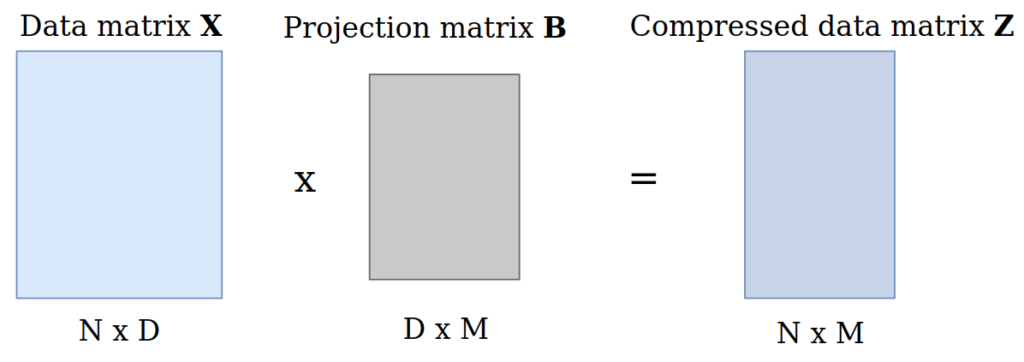### Projection perspective

Another way to derive PCA is to consider the original data points $$\boldsymbol{x}_n$$ and their reconstructions $$\boldsymbol{\tilde{x}}_n$$. In this perspective, we are trying to find reconstructions $$\boldsymbol{\tilde{x}}_n$$ that minimize the averaged squared Euclidean distance between the original datapoints and their reconstructions: $$J = \frac{1}{N} \sum_{n=1}^{N}||\boldsymbol{x}_n – \boldsymbol{\tilde{x}}_n ||^2$$.

You can find more details about this perspective in chapter 10.3 of the book Mathematics for Machine Learning.

## Interpreting the projection matrix

In the beginning, I mentioned that PCA constructs new features (the principal components) that are linear combinations of the original features. Let’s take a closer look at what this means.

Each data point is stored in a vector with $$D$$ elements: $$\boldsymbol{x}_n = [x_{n1}, …, x_{nD}]$$. Each of the $$D$$ dimensions represents a different feature. For example, think of an image with $$D = 64$$ pixels. We can describe each data point as a linear combination of the features:
$$\boldsymbol{x}_n = x_{n1} \cdot \text{feature}_1 + x_{n2} \cdot \text{feature}_2 + … + x_{nD} \cdot \text{feature}_D$$

Using the example of pixels this would correspond to:
$$\boldsymbol{x}_n = x_{n1} \cdot \text{pixel}_1 + x_{n2} \cdot \text{pixel}_2 + … + x_{nD} \cdot \text{pixel}_D$$

When PCA projects the data to a low-dimensional space it also uses a combination of the features. This becomes evident in the projection equation we have seen above:

$$\boldsymbol{z}_n = \boldsymbol{B}^T \boldsymbol{x}_n \in \mathbb{R}^M$$

In this equation, we find the compressed representation $$\boldsymbol{z}_n$$ of the datapoint $$\boldsymbol{x}_n$$ by performing a matrix multiplication. The new, compressed representation of a datapoint $$\boldsymbol{x}_n$$ will be given by a linear combination of all its feature values. We can make this clear when considering an example.

Let’s say our data matrix $$\boldsymbol{X}$$ has 3 datapoints and 2 features, so $$\boldsymbol{X} \in \mathbb{R}^{3×2}$$ and we consider only the first principal component when performing PCA. Hence, we have $$\boldsymbol{B} \in \mathbb{R}^{2×1}$$. When multiplying the data matrix with the projection matrix we receive the compressed versions of the data points as a matrix $$\boldsymbol{Z} \in \mathbb{R}^{3×1}$$. Each one-dimensional code $$\boldsymbol{z}_n$$ is given by a linear combination of the original feature values: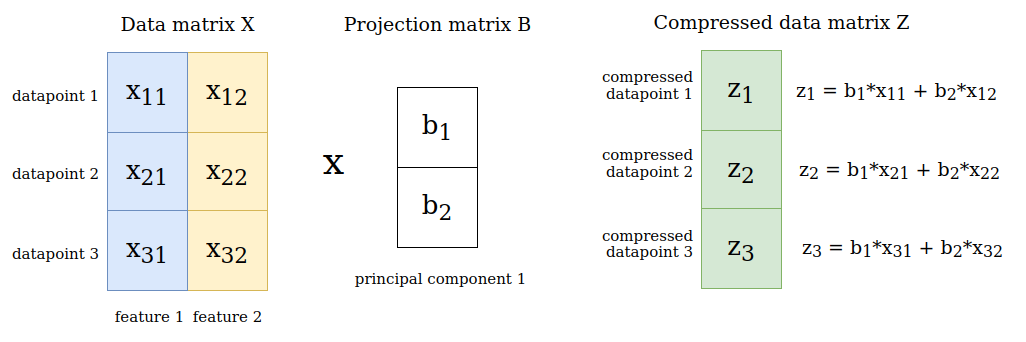## Computing the eigenvectors

By now we know that we have to compute eigenvalues and eigenvectors to perform PCA. We have two ways to do that:

1. Perform an eigendecomposition of the data covariance matrix $$\boldsymbol{S}$$
2. Perform a singular value decomposition of the data matrix $$\boldsymbol{X}$$

The standard approach (which we will use in this tutorial) is the first approach. You can find more on approach two in chapter 10.4 of the Mathematics for Machine Learning book.

Note: In many applications, we only need the first few eigenvectors. Performing a full eigendecomposition or singular value decomposition would be computationally wasteful. Therefore, most software packages implement iterative methods which directly optimize the required number of eigenvectors.

## Summary of important terminology

• $$\boldsymbol{x}_n$$ are our datapoints, stored in the matrix $$\mathcal{\boldsymbol{X}} = \{\boldsymbol{x}_1, …, \boldsymbol{x}_N\}, \boldsymbol{x}_n \in \mathbb{R}^D$$.
• $$\boldsymbol{S}$$ is the data covariance matrix.
• $$\boldsymbol{B}$$ is the projection matrix and consists of column vectors $$\boldsymbol{b}_m$$: $$\boldsymbol{B} := [\boldsymbol{b}_1, …, \boldsymbol{b}_M] \in \mathbb{R}^{D x M}$$.
• $$\boldsymbol{z}_n$$ is the low-dimensional representation of $$\boldsymbol{x}_n$$: $$\boldsymbol{z}_n = \boldsymbol{B}^T \boldsymbol{x}_n \in \mathbb{R}^M$$.

## Implementation

### Dataset

We will use the digits dataset from scikit-learn for our implementation. It contains 8×8 images of handwritten digits (1797 in total). The data is stored in a data matrix with 1797 rows and 64 columns (corresponding to all 8×8=64 pixel values).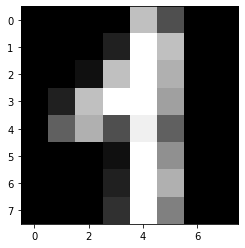### Standardization

Before we can apply PCA, we should standardize the data. If you are wondering why this is necessary, check out this explanation on StackExchange. Standardization rescales the data to have a mean zero and a standard deviation one.

### Computing the data covariance matrix

To find the principal components we first have to compute the data covariance matrix $$\boldsymbol{S}$$:

$$\boldsymbol{S} = \frac{1}{N} \sum_{n=1}^{N} \boldsymbol{x}_n \boldsymbol{x}_n^T$$

We can summarize the summation as follows:

$$\boldsymbol{S} = \frac{1}{N} \boldsymbol{X}^T \boldsymbol{X}$$

### Computing the eigenvalues and eigenvectors

To compute eigenvalues and eigenvectors, we perform an eigendecomposition of the covariance matrix $$\boldsymbol{S}$$. Doing this manually is messy and it is hard to do the computations correctly. Therefore, we will make use of NumPy to get both eigenvalues and eigenvectors. If you want to learn more about how to perform an eigendecomposition, take a look at this Wikipedia article.

### Choosing the number of eigenvalues

In order to decide how many eigenvalues we want to use, we should ask ourselves what our goal is. Remember that the number of eigenvalues that we select corresponds to the dimensionality of the new low-dimensional space we are creating.

Most of the time, PCA is used to reduce the dimensionality of data. But at the same time, we want to make sure that our solution is good, meaning that we do not lose a lot of information. Consequently, we should try to select the eigenvalues that explain most of the variance in the data.

Typically, the first few eigenvalues capture most of the variance whereas later ones do not add much information. When using tools like scikit-learn, we can specify how much variance should be explained by the solution so we do not have to choose the number of eigenvalues ourselves.

In our case, we can decide how many principal components to use by computing the explained variance of the individual eigenvectors.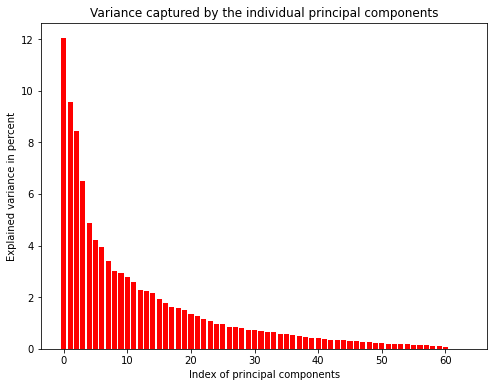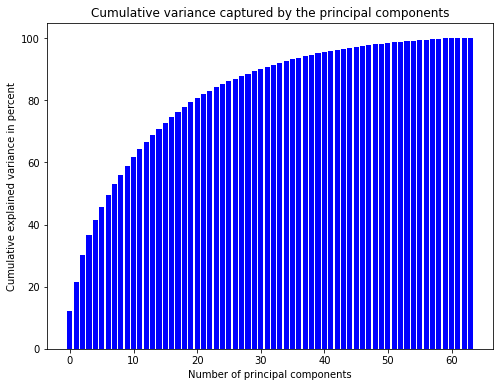The left plot shows that the first principal components explain more variance than the latter ones. However, the amount of variance explained is not very high. Consequently, we would lose a lot of information when using only the first few principal components. The plot to the right shows that we would have to keep 31 principal components to explain 90% of the variance. This is a lot but still better than keeping all 64 values.

For the purpose of this tutorial, we will keep only the 2 largest eigenvalues and their corresponding eigenvectors. This will allow us to visualize the result in two dimensions.

### Constructing the projection matrix

After finding the eigenvectors/principal components, we can arrange them into a matrix (our projection matrix $$\boldsymbol{B}$$).

### Projecting the data

In order to actually project the original data $$\boldsymbol{X}$$ into the lower-dimensional space, we multiply it with the matrix $$\boldsymbol{B}$$:

$$\boldsymbol{Z} = \boldsymbol{X} \boldsymbol{B} \in \mathbb{R}^{N x M}$$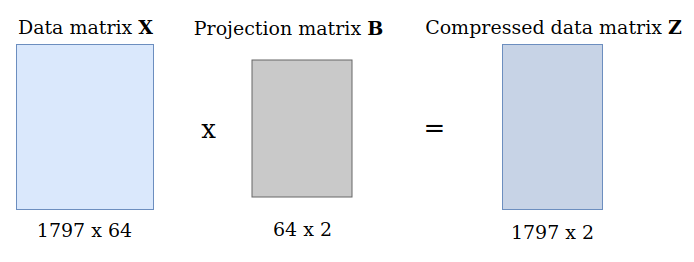### Plotting the transformed data

With only two principal components, we can plot the transformed data easily. One axis will correspond to the first principle component, the other axis to the second.

### Comparison to scikit-learn

In practice, it does not make sense to perform PCA manually. Instead, we can use packages like scikit-learn. This simplifies the computation substantially. Let’s apply principal component analysis as contained in scikit-learn to the digits dataset and compare it to our solution. As visible in the plot below, our implementation of PCA gives the same result as the scikit-learn version!

##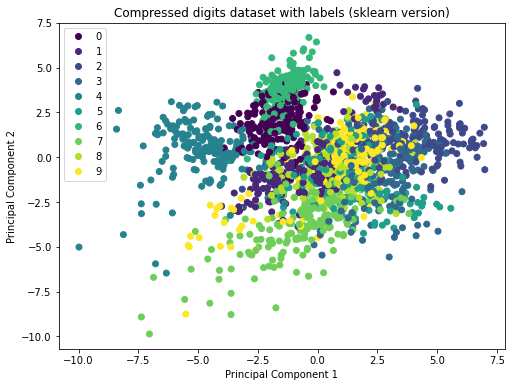The basis for this notebook is chapter 10 of the book Mathematics for Machine Learning. I can highly recommend reading through the chapter to get a deeper understanding of PCA.

Another fantastic explanation of PCA is given in this post on StackExchange.

## One thought on “Dimensionality Reduction with Principal Component Analysis”

1.ZA sagt:

Sometimes study through cases can be very beneficial for understanding.

An interesting case-study of PCA can be found here:
https://iopscience.iop.org/article/10.1088/2632-2153/abd87c

This is the case where PCA was applied in conjunction with the K-means clustering to perform an advanced characterization of monolayer matter, where different physically distinct areas were identified.